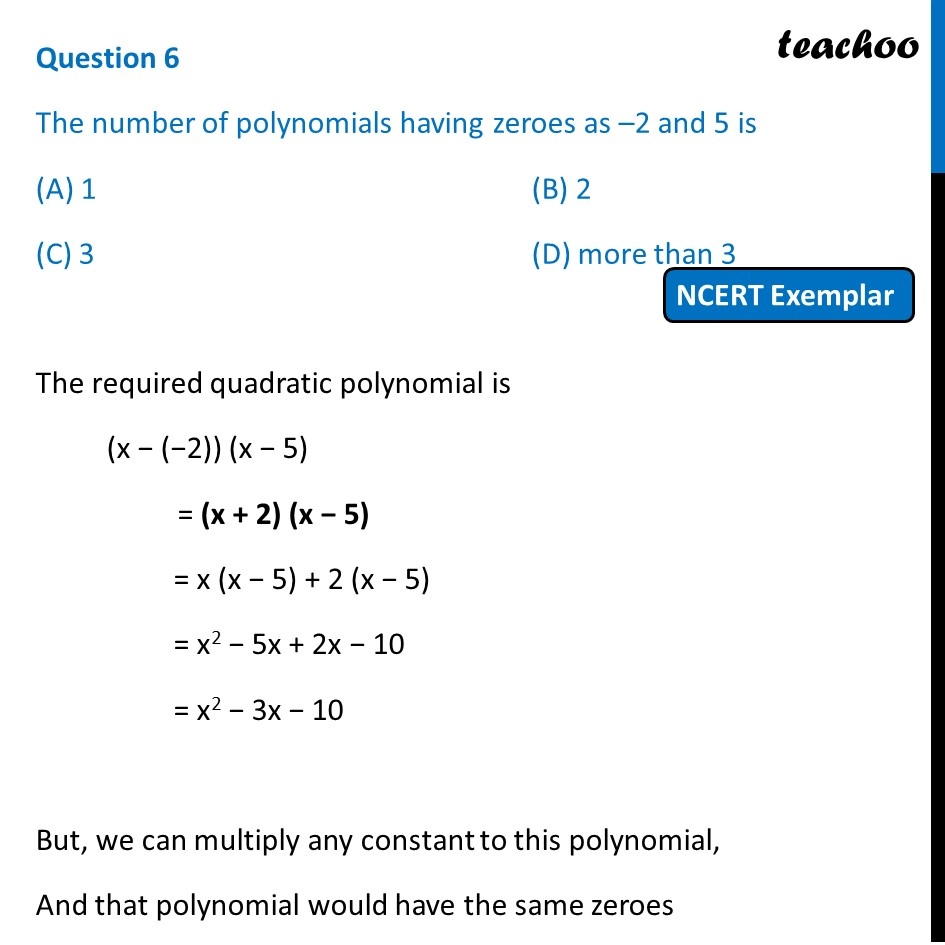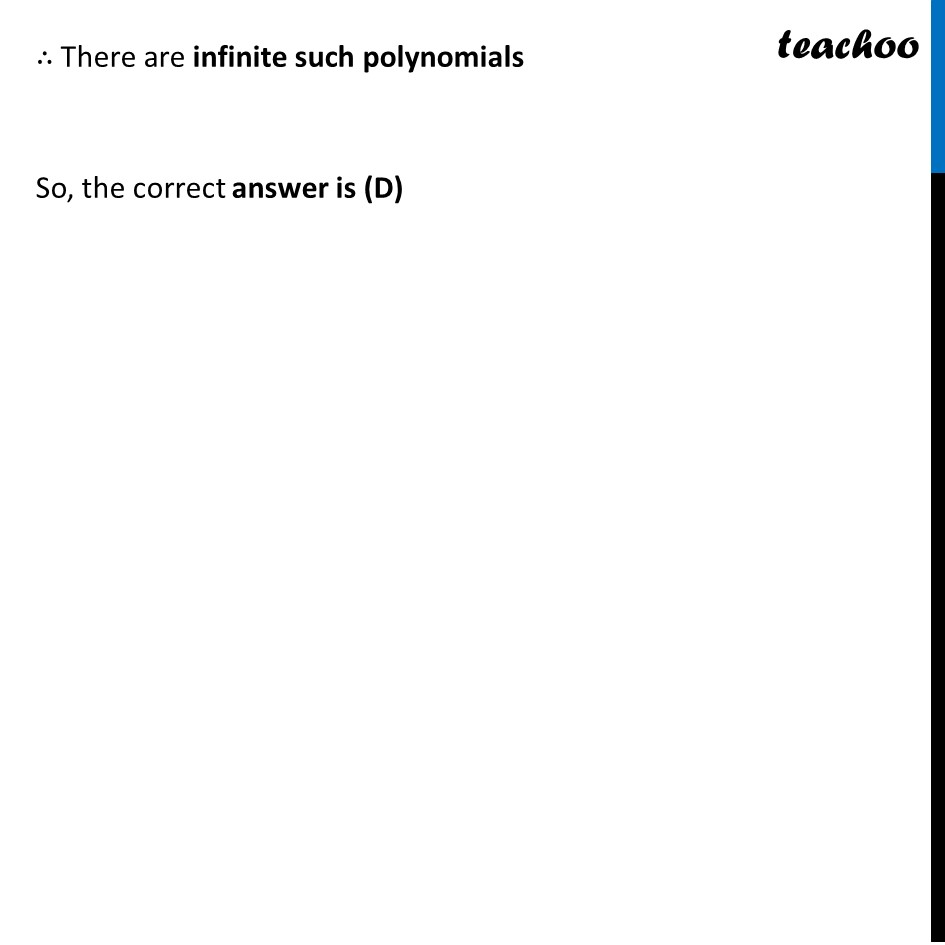## (C) 3                               (D) more than 31. Chapter 2 Class 10 Polynomials (Term 1)
2. Serial order wise
3. MCQs from NCERT Exemplar

Transcript

Question 6 The number of polynomials having zeroes as –2 and 5 is (A) 1 (B) 2 (C) 3 (D) more than 3 The required quadratic polynomial is (x − (−2)) (x − 5) = (x + 2) (x − 5) = x (x − 5) + 2 (x − 5) = x2 − 5x + 2x − 10 = x2 − 3x − 10 But, we can multiply any constant to this polynomial, And that polynomial would have the same zeroes ∴ There are infinite such polynomials So, the correct answer is (D)

MCQs from NCERT Exemplar